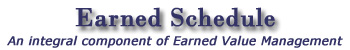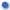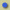The Earned Schedule (ES) calculators are freely available and downloadable from this site.  There are two Excel spreadsheets.  The one most used (v1) does not account for partial periods, that is, the first period and the last.  The second version (v2) does account for partial periods.

The v1 calculator assumes in the calculations that all periods are full and not partial.  Once several periods are involved in the calculation, the error introduced becomes negligible.  For those that are applying ES to weekly Earned Value, the error is small from the outset.

For a discussion of the error in the ES calculation please reference the Presentations page for the .pdf file, “Earned Schedule Training,” 17th IIPMC, Nov 7-9, 2005.  The section “Interpolation Error” discusses the partial period along with other sources of error.ES Calculator v1b (does not account for partial periods)ES Calculator v2c (does account for partial periods)

The special case ES calculator is intended for small projects having downtime and stop work conditions. The calculator produces special indicators and forecasts. Comparison of the special to uncorrected ES forecast is made available.ES Calculator vs1f (Special Cases)

The Earned Schedule-Longest Path calculator assists in making the longest path forecasts. It is very similar to the basic ES calculator, and is easy to apply. To determine the longest path, forecasts are to be made for all network paths of the schedule which conclude at project completion. For each status point, the path having the longest duration forecast is taken to best represent the project.ES-LP Calculator v1e

The ES re-plan calculator is useful when a project has undergone significant changes to its PMB. The forecast of total duration for the project is computed by adding the accrued time duration from project start through the re-plan to the forecast for the project portion remaining. The time-based indicators represent performance after the re-plan.ES Re-Plan Calculator v1

The Milestone SV(t) Forecast calculator is useful for assessing whether a project milestone or significant event is achievable. To effectively use the calculator requires the creation of the PMB using the tasks associated with the milestone. Output of the calculator is computed values for the schedule variance forecast to the milestone date, F-SV(t), and the milestone to complete index, TSPIM.Milestone SV(t) Forecast v1

The P-Factor calculator is a set of Excel spreadsheets.  From the baseline schedule and actual performance, the calculator provides a numerical indicator of how well the schedule is followed, i.e. a measure of “schedule adherence.” An output from the calculator useful to management is the identification of tasks which may be experiencing constraints or impediments.P-Factor Calculator v2

The Schedule Adherence and Rework Calculator utilizes the periodic values for the P-factor and the EV accrued to compute and graph the EV accomplished out of sequence and the rework it causes.SAI, Rework & Duration Increase Calculator v3a

The Prediction Analysis Calculator identifies the likelihood that the project will complete at a desired time (ED). The spreadsheet determines the point at which the project exceeds the threshold and becomes “out of control.” The calculator is useful for assessing the opportunity for project recovery and having a successful outcome. As well, the calculator assists with creating a reasonable recovery strategy.Prediction Analysis Calculator v2b

The Probability of Recovery Calculator identifies whether the threshold values for TSPI and TCPI have been exceeded. Also provided in the output is the period of opportunity for recovery as well as the probability of recovery for both project cost and duration. The calculator is very easy to apply, needing only EVM and ES data normally available.Probability of Recovery Calculator v1a

Statistical Forecasting Calculator computes high and low confidence limits for final cost and schedule duration. The calculator is reasonably simple to apply. The user does not require in-depth understanding of statistics.Statistical Forecasting Calculator v3b
 Application Instructions Example – Late Finish, daily status

The Forecasting Method Selector Calculator provides duration forecasts with improved accuracy, by appropriately selecting between two duration forecasting formulas: IEAC(t) = PD/SPI(t) or IEAC(t) = AT + (PD – ES).Forecasting Method Selector Calculator v1a

Statistical Planning Calculator (Probability & Reserves) is intended to assist planning teams for strategizing the amounts of cost and schedule reserve needed to achieve a desired probability of success.  Use of the calculator requires either estimates or historical variation from past projects.Statistical Planning Calculator

The Stability Point Calculator determines the observation number in a sequence of CPI and SPI(t) values at which all subsequent observations are within a defined stability limit.Stability Point CalculatorIntroductory VideoConcept DescriptionES Enhances EVMEVM Time ForecastingContactsTraining SourcesSites of InterestEVM - ES ToolsES BooksES Book (Translations)ES AwardsCopyrights & TrademarksPCA Interview
 Copyright © 2006 Walt Lipke